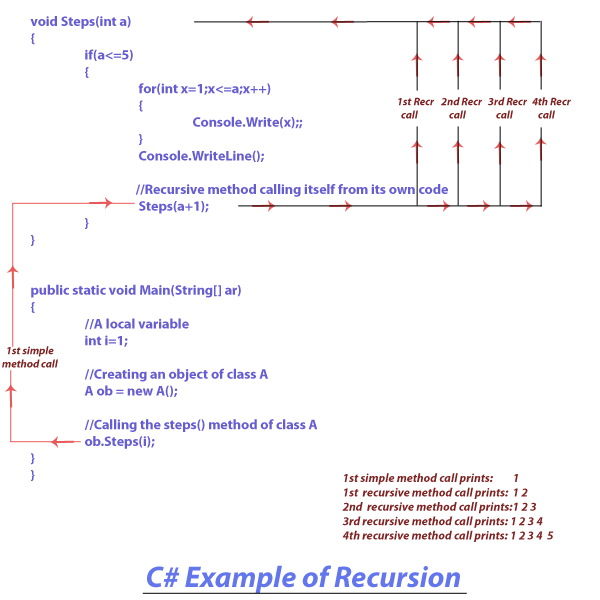< Prev
Next >

# C# Recursion

In C# language, we can execute a technique by which a method will call itself to do a specific task, which is known as recursion. The method that can call itself from its own code is said to be a "recursive method".

To understand the technique of recursion, we will create two programs to do the same task of printing a numbers from 1 to 5 in successive steps. For example -
• Printing 1 in the first line.
• Printing 1 and 2 in the second line.
• Printing 1, 2 and 3 in the third line.
• Printing 1, 2, 3 and 4 in the fourth line
• Printing 1, 2, 3, 4 and 5 in the fifth line.

In the first program, we will see a simple method(a non-recursive method) doing the task and in the second program we will implement a recursive method doing the same task.

## A simple method(non-recursive) to print numbers from 1 to 5 in steps

Let's see the first program which implements a simple non-recursive method, which has used two for loops(one nested in another) to implement the task of printing the numbers from 1 to 5 in steps, as mentioned above.

``````//C# Example of a simple(non-recursive) method

using System;

class A
{
//Defining the Steps() method of class A
void Steps(int a)
{
for(int x=a;x<=5;x++)
{
for(int y=1;y<=x;y++)
{
Console.Write(y);
}
Console.WriteLine();
}
}

//Defining the Main() method of class A
public static void Main(String[] ar)
{
//A local variable
int i=1;

//Creating an object of class A
A ob = new A();

//Calling the steps() method of class A
ob.Steps(i);
}
}``````

## Output

``````1
1 2
1 2 3
1 2 3 4
1 2 3 4 5``````

## Recursive method to print numbers from 1 to 5 in steps

Let's see the second program that creates a recursive method with the name steps, which calls itself to print the numbers from 1 to 5 in successive steps.

``````//C# Example of a recursive method

using System;

class A
{
//Defining a method of class A
void Steps(int a)
{
if(a<=5)
{
for(int x=1;x<=a;x++)
{
Console.Write(x);;
}
Console.WriteLine();

//Recursive method calling itself from its own code
Steps(a+1);
}
}

//Defining a method of class A
public static void Main(String[] ar)
{
//A local variable
int i=1;

//Creating an object of class A
A ob = new A();

//Calling the steps() method of class A
ob.Steps(i);
}
}``````

## Output

``````1
1 2
1 2 3
1 2 3 4
1 2 3 4 5``````

## Program Analysis

Let us understand our recursion program with a diagram -As you can see in the self-explanatory diagram, the method steps() is called in successions, like -
• Starting the program, the Main() method calls method Steps(), which prints 1.
• In the next call, the Steps() method calls itself recursively for the first time, which prints 1,2
• During the next call, the Steps() method calls itself recursively for the second time, which prints 1,2,3
• Next, the Steps() method calls itself recursively for the third time, which prints 1,2,3,4
• Finally, the Steps() method calls itself recursively for the fourth time, which prints 1,2,3,4,5

## Another example of Recursion

In the upcoming code example, we are going to find the factorial of 5, by using a recursive method that calls itself.

``````//C# Recursion Example - Finding Factorial

using System;

class A
{
public static void Main(String[] ar)
{
//Creating an object of class A
A ob = new A();

//Local variable whose factorial is to be found
int num = 5;

//Calling the recursive method to calculate a factorial of an int local variable
int result = ob.Factor(num);

//Printig the result of factorial
Console.WriteLine("Factorial of 5 is " + result);
}

//Defining a recursive method of class A
int Factor(int a)
{
//Local variable
int result;

if(a==1)
return 1;
else
result = a * Factor(a-1); // recursive method factor has called itself

//Returning the final result
return result;
}
}``````

## Output

``Factorial of 5 is 120``